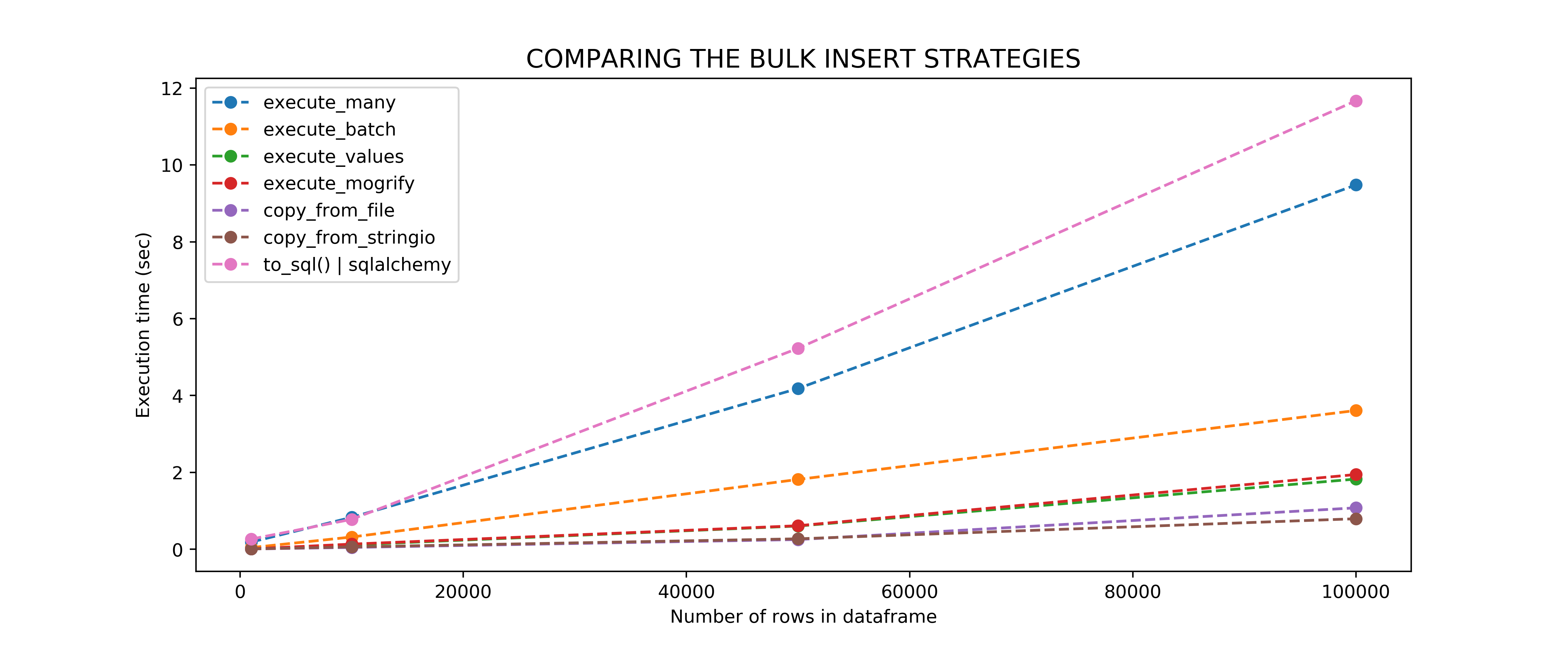# Pandas to PostgreSQL using Psycopg2: Bulk Insert Performance Benchmark

If you have ever tried to insert a relatively large dataframe into a PostgreSQL table, you know that single inserts are to be avoided at all costs because of how long they take to execute.  There are multiple ways to do bulk inserts with Psycopg2 (see this Stack Overflow page and this blog post for instance). It becomes confusing to identify which one is the most efficient. In this post, I compared the following 7 bulk insert methods, and  ran the benchmarks for you:

• execute_many()
• execute_batch()
• execute_values() – view post
• mogrify() then execute() – view post
• copy_from() – there are two ways to do this – view post
• to_sql() via sqlalchemy

For a fully functioning tutorial on how to replicate this, please refer to my Jupyter notebook on GitHub.

### Step 1: Specify the connection parameters

```# Here you want to change your database, username & password according to your own values
param_dic = {
"host"      : "localhost",
"database"  : "globaldata",
"user"      : "myuser",
}```

### Step 2: Load the pandas dataframe, and connect to the database

The data for this tutorial is freely available on https://datahub.io/core/global-temp, but you will also find it in the data/ directory of my GitHub repository. What is nice about this dataframe is that it contains string, date and float columns, so it should be a good test dataframe for bench-marking bulk inserts.

```import pandas as pd

csv_file = "../data/global-temp-monthly.csv"
df = df.rename(columns={
"Source": "source",
"Date": "datetime",
"Mean": "mean_temp"
})
```
```def connect(params_dic):
""" Connect to the PostgreSQL database server """
conn = None
try:
# connect to the PostgreSQL server
print('Connecting to the PostgreSQL database...')
conn = psycopg2.connect(**params_dic)
except (Exception, psycopg2.DatabaseError) as error:
print(error)
sys.exit(1)
print("Connection successful")
return conn

conn = connect(param_dic)
```

### Step 3: The seven different ways to do a Bulk Insert using Psycopg2

You are welcome.

```import os
import psycopg2
import numpy as np
import psycopg2.extras as extras
from io import StringIO

def execute_many(conn, df, table):
"""
Using cursor.executemany() to insert the dataframe
"""
# Create a list of tupples from the dataframe values
tuples = [tuple(x) for x in df.to_numpy()]
# Comma-separated dataframe columns
cols = ','.join(list(df.columns))
# SQL quert to execute
query  = "INSERT INTO %s(%s) VALUES(%%s,%%s,%%s)" % (table, cols)
cursor = conn.cursor()
try:
cursor.executemany(query, tuples)
conn.commit()
except (Exception, psycopg2.DatabaseError) as error:
print("Error: %s" % error)
conn.rollback()
cursor.close()
return 1
print("execute_many() done")
cursor.close()

def execute_batch(conn, df, table, page_size=100):
"""
Using psycopg2.extras.execute_batch() to insert the dataframe
"""
# Create a list of tupples from the dataframe values
tuples = [tuple(x) for x in df.to_numpy()]
# Comma-separated dataframe columns
cols = ','.join(list(df.columns))
# SQL quert to execute
query  = "INSERT INTO %s(%s) VALUES(%%s,%%s,%%s)" % (table, cols)
cursor = conn.cursor()
try:
extras.execute_batch(cursor, query, tuples, page_size)
conn.commit()
except (Exception, psycopg2.DatabaseError) as error:
print("Error: %s" % error)
conn.rollback()
cursor.close()
return 1
print("execute_batch() done")
cursor.close()

def execute_values(conn, df, table):
"""
Using psycopg2.extras.execute_values() to insert the dataframe
"""
# Create a list of tupples from the dataframe values
tuples = [tuple(x) for x in df.to_numpy()]
# Comma-separated dataframe columns
cols = ','.join(list(df.columns))
# SQL quert to execute
query  = "INSERT INTO %s(%s) VALUES %%s" % (table, cols)
cursor = conn.cursor()
try:
extras.execute_values(cursor, query, tuples)
conn.commit()
except (Exception, psycopg2.DatabaseError) as error:
print("Error: %s" % error)
conn.rollback()
cursor.close()
return 1
print("execute_values() done")
cursor.close()

def execute_mogrify(conn, df, table):
"""
Using cursor.mogrify() to build the bulk insert query
then cursor.execute() to execute the query
"""
# Create a list of tupples from the dataframe values
tuples = [tuple(x) for x in df.to_numpy()]
# Comma-separated dataframe columns
cols = ','.join(list(df.columns))
# SQL quert to execute
cursor = conn.cursor()
values = [cursor.mogrify("(%s,%s,%s)", tup).decode('utf8') for tup in tuples]
query  = "INSERT INTO %s(%s) VALUES " % (table, cols) + ",".join(values)

try:
cursor.execute(query, tuples)
conn.commit()
except (Exception, psycopg2.DatabaseError) as error:
print("Error: %s" % error)
conn.rollback()
cursor.close()
return 1
print("execute_mogrify() done")
cursor.close()

def copy_from_file(conn, df, table):
"""
Here we are going save the dataframe on disk as
a csv file, load the csv file
and use copy_from() to copy it to the table
"""
# Save the dataframe to disk
tmp_df = "./tmp_dataframe.csv"
f = open(tmp_df, 'r')
cursor = conn.cursor()
try:
cursor.copy_from(f, table, sep=",")
conn.commit()
except (Exception, psycopg2.DatabaseError) as error:
os.remove(tmp_df)
print("Error: %s" % error)
conn.rollback()
cursor.close()
return 1
print("copy_from_file() done")
cursor.close()
os.remove(tmp_df)

def copy_from_stringio(conn, df, table):
"""
Here we are going save the dataframe in memory
and use copy_from() to copy it to the table
"""
# save dataframe to an in memory buffer
buffer = StringIO()
buffer.seek(0)

cursor = conn.cursor()
try:
cursor.copy_from(buffer, table, sep=",")
conn.commit()
except (Exception, psycopg2.DatabaseError) as error:
print("Error: %s" % error)
conn.rollback()
cursor.close()
return 1
print("copy_from_stringio() done")
cursor.close()

#----------------------------------------------------------------
# SqlAlchemy Only
#----------------------------------------------------------------
from sqlalchemy import create_engine

connect = "postgresql+psycopg2://%s:%s@%s:5432/%s" % (
param_dic['user'],
param_dic['host'],
param_dic['database']
)

def to_alchemy(df):
"""
Using a dummy table to test this call library
"""
engine = create_engine(connect)
df.to_sql(
'test_table',
con=engine,
index=False,
if_exists='replace'
)
print("to_sql() done (sqlalchemy)")

```

### Step 4: Performance benchmark

For the details on how the benchmarking was done, please refer to the ‘Benchmarking’ section of this notebookSo execute_values() and execute_mogrify() are doing fine…but the fastest way is to use copy_from(). You can either

• save your dataframe to a stringio object and load it directly to SQL, or
• save your dataframe to disk and loas it to SQL

I don’t know about you, but it is kind of sad to see that a good old copy is doing a better job than execute_values() and execute_mogrify()… But sometimes low tech is best.  Just like when you try to reach your colleague through Google Hangouts, Zoom, Skype… and then end up picking your phone and just calling them because the voice is better🙂